Next: Worked Example 2: Lenz's Up: Magnetic Induction Previous: Worked Examples

## Example 9.1: Faraday's law

Question: A plane circular loop of conducting wire of radius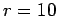cm which possesses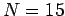turns is placed in a uniform magnetic field. The direction of the magnetic field makes an angle of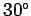with respect to the normal direction to the loop. The magnetic field-strengthis increased at a constant rate from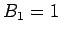T to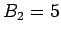T in a time interval of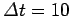s. What is the emf generated around the loop? If the electrical resistance of the loop is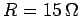, what current flows around the loop as the magnetic field is increased?

Answer: The area of the loop is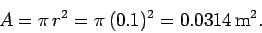The component of the magnetic field perpendicular to the loop is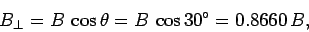whereis the magnetic field-strength. Thus, the initial magnetic flux linking the loop is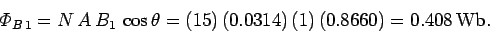Likewise, the final flux linking the loop is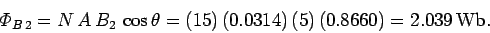The time rate of change of the flux is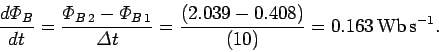Thus, the emf generated around the loop is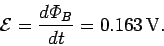Note, incidentally, that one weber per second is equivalent to one volt.

According to Ohm's law, the current which flows around the loop in response to the emf is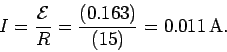Next: Worked Example 2: Lenz's Up: Magnetic Induction Previous: Worked Examples
Richard Fitzpatrick 2007-07-14